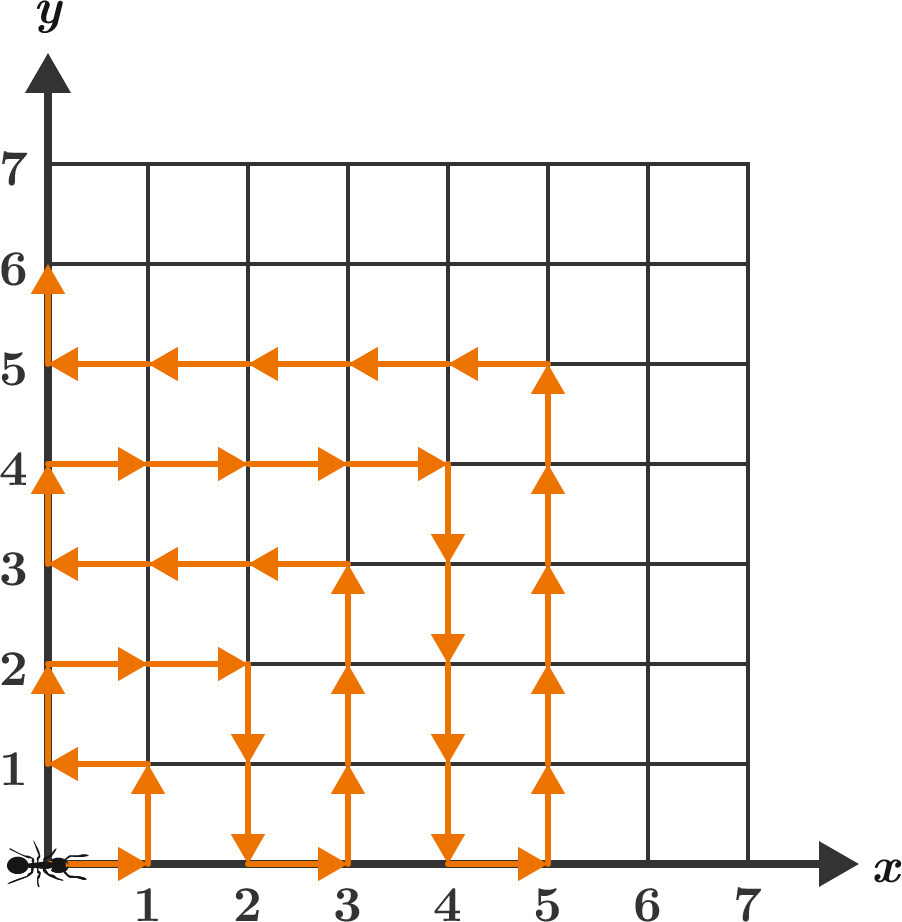# Where is the ant after 2018 steps?

An ant begins from $(0,0)$ in the $xy$-coordinate plane. It then travels to each point with non-negative integer coordinates following the pattern shown below.

If the ant stops moving after $2018$ units, find its final position $(x,y)$ and enter your answer as $x+y.$×